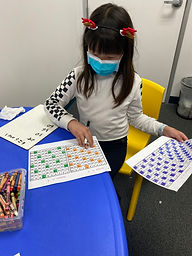Ms. Briana

Target 1​

Lesson Type:

Continuation

Number Operation

:

Integer Composition

Recognize a whole number is a multiple of each of its factors.

1:

Determine whether a given whole number in the range of one to 100 is a multiple of a given one-digit number.

2:

Using a hundred’s chart, identify all the multiples of two through ten.

3:

Recognize patterns in multiples, setting the foundation for developing computational fluency in multiplication.

4th

Vocabulary:

Multiples, Factors

Activities:

Students highlighted multiples of 3,4,6,7 on 100 chart and discussed what they noticed and patterns.

Students worked together to create factor flowers on the board with the help of their 100's chart.Home Exploration

Guiding Questions:Absent Students:

Target 2

:

1:

Define perimeter.

2:

Understand that perimeter is the distance around a figure.

3:

Understand that perimeter can be found by adding the lengths of the sides of a figure.

3rd

Vocabulary:

Tailors Tape, Perimeter

Activities:

Students were given a holiday tree print out and they used rulers and tailors tape to measure the length of all the sides.

Students then used those measurement to determine the perimeter of the tree.Home Exploration

Guiding Questions:Target 3

:

Vocabulary:

Activities:Home Exploration